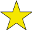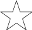Advertisements

## Cryptograms (#1)

Difficulty:(explanation of difficulty)

A cryptogram is a short piece of text that has been encrypted in substitution code. For example, the phrase "HOW NOW BROWN COW" could become "CLZ WLZ HGLZW RLZ" if C were substituted for H, L for O, Z for W, and so on. Your task is to break the code. To do this, you can look for common letters and/or words. For example, a letter that appears more often than any other is likely to represent E or T; a one-letter word is likely to be A (although it could be I); a three-letter word repeated multiple times is likely to be THE or AND; a letter that is doubled in a word is more likely to be E or T than A or H; and so on. The code is different for each cryptogram on this page.

Instructions: When you determine which letter an encrypted letter represents, enter the unencrypted letter in the box to the right of "encrypted letter becomes", where encrypted letter is the encrypted letter. The decrypted letter will then appear above each occurrence of the encrypted letter. Continue doing so until all of the squares are filled in for the cryptogram in question.

 U T O M L T Q C Z D G L D Z C L O N O L J Y H L Q Z F B D T O Y , Z D G B O N Y Y F N I B V Y Z D C L T Q . N B O X B O C Z U Q Y O O M W B Q I H L Q Z D C L T Q , Z D G B O N Y Y F N I B V Y Z F B D T O Y . O C Z O ' N Q Y I Z O B J B O M . ( Z I A Y Q O Y B D N O Y B D )
A becomes: B becomes: C becomes: D becomes: F becomes: G becomes: H becomes: I becomes: J becomes: L becomes: M becomes: N becomes: O becomes: Q becomes: T becomes: U becomes: V becomes: W becomes: X becomes: Y becomes: Z becomes:
 A G C W S A G C J A Q J U C F A S M T G C J W E K D G E C B S Q K K S P , " E I F S Q J I L A G C J D S Q M T K C C F S Q P S N , G C N S Q M T A Q J P S B C J E P G E K O J L B F . "
A becomes: B becomes: C becomes: D becomes: E becomes: F becomes: G becomes: I becomes: J becomes: K becomes: L becomes: M becomes: N becomes: O becomes: P becomes: Q becomes: S becomes: T becomes: U becomes: W becomes:
 V N I D T D M O I R I V U E Y Z U D N N V A R , E Z O O I V O D N M Y O X I V O N I D T N V U R E Z D J O A Y U .
A becomes: D becomes: E becomes: I becomes: J becomes: M becomes: N becomes: O becomes: R becomes: T becomes: U becomes: V becomes: X becomes: Y becomes: Z becomes:
 E D S U I D S M Y Q I H D O V O V E X D D Y M T X Q D S Q X D H D F T H Q O D I D S H U .
D becomes: E becomes: F becomes: H becomes: I becomes: M becomes: O becomes: Q becomes: S becomes: T becomes: U becomes: V becomes: X becomes: Y becomes:
 G R T K Y X S P T U Y M S G H G R T U X Z N N T A , " M G ' U S T P M F R G N Z P R Y W M X F L H Z H W T A . "
A becomes: F becomes: G becomes: H becomes: K becomes: L becomes: M becomes: N becomes: P becomes: R becomes: S becomes: T becomes: U becomes: W becomes: X becomes: Y becomes: Z becomes: